#Impulse Functions: Dirac FunctionIt is very common for physical problems to have impulse behavior, large quantities acting over very short periods of time. These kinds of problems often lead to differential equations where the nonhomogeneous term g(t) is very large over a small interval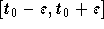and is zero otherwise. The total impulse of g(t) is defined by the integral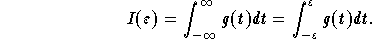In particular, let us assume that g(t) is given by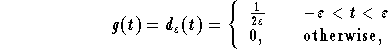where the constantis small. It is easy to see that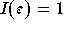. When the constantbecomes very small the value of the integral will not change. In other words,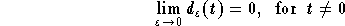,

while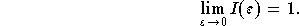This will help us define the so-called Dirac delta-function by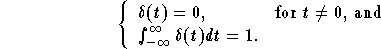If we put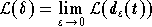, then we have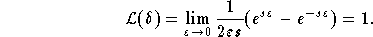More generally, we have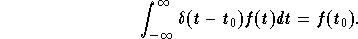Example: Find the solution of the IVP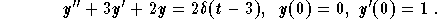(1)
We apply the Laplace transform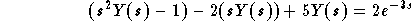,

where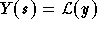. Hence,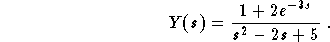;

(2)
Inverse Laplace:

Since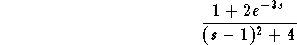,

and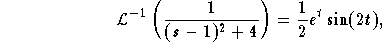we get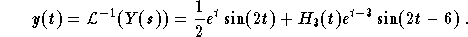[Differential Equations] [First Order D.E.] [Second Order D.E.]
[Geometry] [Algebra] [Trigonometry ]
[Calculus] [Complex Variables] [Matrix Algebra]S.O.S MATHematics home page

Do you need more help? Please post your question on our S.O.S. Mathematics CyberBoard.Author: Mohamed Amine Khamsi